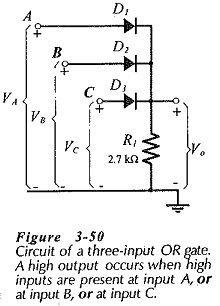## Diode Logic Circuits:

A Diode Logic Circuits produces an output voltage which is either high or low, depending upon the levels of several input voltages. The two basic logic circuits are the AND gate and the OR gate.

### Diode AND Gate:Figure 3-49 shows the circuit diagram of a diode AND gate. It is seen that the diode anodes are connected to a 5 V supply (VCC) via resistor R1. The circuit has a single output terminal at the diode anodes, and three input terminals at the device cathodes. (An AND gate could have any number of inputs from 2 up to perhaps 50.)

If one (or more) of the input terminals is grounded, current flows from the supply through R1 and through the forward-biased diode to ground. In this case, the output voltage is just VF above ground, (0.7 V for silicon). The output is said to be at a low level. When input levels of 5 V are applied to all three input terminals, none of the diodes is forward biased, no resistor current flows, and no significant voltage drop occurs across R1. Thus, the output voltage is equal to VS, and it is referred to as a high output level.

An AND gate produces a high output only when input A is high. AND input B is high, AND input C is high.

### Diode OR Gate:

The circuit diagram of an OR gate with three input terminals is shown in Fig. 3-50 Like the AND gate, the OR gate could have two or more inputs. It is obvious that the output voltage (Vo) of the OR gate in Fig. 3-50 is low when all three inputs are low. Now suppose that a +5 V input is applied to terminal A, while terminals B and C remain grounded. Diode D1 becomes forward biased, and its cathode voltage is Vo = (5 V – VF). The gate output voltage is high, and diodes D2 and D3 are reverse biased with +Vo at the cathodes and ground at the anodes. The output will be high if a high input voltage is applied to any one (or more) of the inputs.An OR gate produces a high output when input A is high, OR or when input B is high, OR when input C is high.

Diode AND and OR gates can be designed and constructed using discrete components. However, integrated-circuit (IC) packages are available containing many Diode Logic Circuits already fabricated in the form of one or more gates.

Scroll to Top